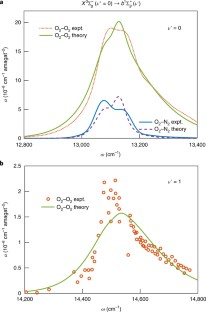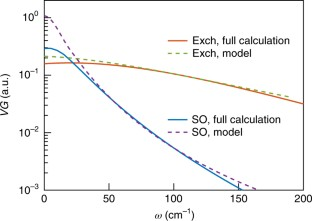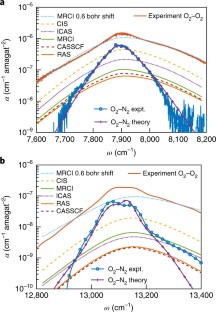Thank you for visiting nature.com. You are using a browser version with limited support for CSS. To obtain the best experience, we recommend you use a more up to date browser (or turn off compatibility mode in Internet Explorer). In the meantime, to ensure continued support, we are displaying the site without styles and JavaScript.

# O2−O2 and O2−N2 collision-induced absorption mechanisms unravelled

### Subjects

A Publisher Correction to this article was published on 13 April 2018

## Abstract

Collision-induced absorption is the phenomenon in which interactions between colliding molecules lead to absorption of light, even for transitions that are forbidden for the isolated molecules. Collision-induced absorption contributes to the atmospheric heat balance and is important for the electronic excitations of O2 that are used for remote sensing. Here, we present a theoretical study of five vibronic transitions in O2−O2 and O2−N2, using analytical models and numerical quantum scattering calculations. We unambiguously identify the underlying absorption mechanism, which is shown to depend explicitly on the collision partner—contrary to textbook knowledge. This explains experimentally observed qualitative differences between O2−O2 and O2−N2 collisions in the overall intensity, line shape and vibrational dependence of the absorption spectrum. It is shown that these results can be used to discriminate between conflicting experimental data and even to identify unphysical results, thus impacting future experimental studies and atmospheric applications.

## Access options

from\$8.99

All prices are NET prices.Fig. 1: Experimental and theoretical collision-induced absorption spectra for the $${X}^{3}{\Sigma }_{g}^{-}(v^{\prime\prime} =0)\to {a}^{1}{\Delta }_{g}(v^{\prime} )$$ bands of O2−O2 and O2−N2.Fig. 2: Experimental and theoretical collision-induced absorption spectra for the $${X}^{3}{{\rm{\Sigma }}}_{g}^{-}(v^{\prime\prime} =0)\to {b}^{1}{{\rm{\Sigma }}}_{g}^{+}(v^{\prime} )$$ bands of O2−O2 and O2−N2.Fig. 3: Translational profiles for the $${X}^{3}{\Sigma }_{g}^{-}\to {a}^{1}{{\rm{\Delta }}}_{g}$$ transition for both exchange and spin–orbit mechanisms.Fig. 4: Collision-induced absorption spectra for the $${X}^{3}{{\rm{\Sigma }}}_{g}^{-}(v^{\prime\prime} =0)\to {b}^{1}{{\rm{\Sigma }}}_{g}^{+}(v^{\prime} =0)$$ band in air, normalized to the product of O2 and air number densities.Fig. 5: Collision-induced absorption spectra for the $${X}^{3}{\Sigma }_{g}^{-}\to {a}^{1}{\Delta }_{g}$$ and $${b}^{1}{{\rm{\Sigma }}}_{g}^{+}$$ transitions in O2−O2 and experimental results.

## Change history

• ### 13 April 2018

In the version of this Article originally published, Figures 3 and 4 were erroneously swapped, this has been corrected in all versions of the Article.

## References

1. 1.

Frommhold, L. Collision-Induced Absorption in Gases (Cambridge Univ. Press, Cambridge, 1994).

2. 2.

Crawford, M. F., Welsh, H. L. & Locke, J. L. Infra-red absorption of oxygen and nitrogen induced by intermolecular forces. Phys. Rev. 75, 1607–1607 (1949).

3. 3.

Smith, K. M. & Newnham, D. A. Near-infrared absorption cross sections and integrated absorption intensities of molecular oxygen (O2, O2–O2, and O2–N2). J. Geophys. Res. Atmos. 105, 7383–7396 (2000).

4. 4.

Maté, B., Lugez, C., Fraser, G. T. & Lafferty, W. J. Absolute intensities for the O2 1.27 μm continuum absorption. J. Geophys. Res. Atmos. 104, 30585–30590 (1999).

5. 5.

Long, D. A., Robichaud, D. J. & Hodges, J. T. Frequency-stabilized cavity ring-down spectroscopy measurements of line mixing and collision-induced absorption in the O2 A-band. J. Chem. Phys. 137, 014307 (2012).

6. 6.

Spiering, F. R. et al. Line mixing and collision induced absorption in the oxygen A-band using cavity ring-down spectroscopy. J. Chem. Phys. 133, 114305 (2010).

7. 7.

Spiering, F. R., Kiseleva, M. B., Filippov, N. N., van Kesteren, L. & van der Zande, W. J. Collision-induced absorption in the O2 B-band region near 670 nm. Phys. Chem. Chem. Phys. 13, 9616–9621 (2011).

8. 8.

Spiering, F. R. et al. The effect of collisions with nitrogen on absorption by oxygen in the A-band using cavity ring-down spectroscopy. Mol. Phys. 109, 535–542 (2011).

9. 9.

Spiering, F. R. & van der Zande, W. J. Collision induced absorption in the a 1Δ(v = 2) ← X3 $${}^{{{\rm{\Sigma }}}_{g}^{-}}$$ (v = 0) band of molecular oxygen. Phys. Chem. Chem. Phys. 14, 9923–9928 (2012).

10. 10.

Sneep, M. & Ubachs, W. Cavity ring-down measurement of the O2–O2 collision-induced absorption resonance at 477 nm at sub-atmospheric pressures. J. Quant. Spectrosc. Radiat. Transf. 78, 171–178 (2003).

11. 11.

Sneep, M. & Ubachs, W. in Weakly Interacting Molecular Pairs: Unconventional Absorbers of Radiation in the Atmosphere Vol. 27 (eds Camy-Peret, C. & Vigasin, A.) 203–211 (NATO Science Series: IV: Earth and Environmental Sciences, Springer, 2003).

12. 12.

Tran, H., Boulet, C. & Hartmann, J.-M. Line mixing and collision-induced absorption by oxygen in the A-band: laboratory measurements, model, and tools for atmospheric spectra computations. J. Geophys. Res. 111, D15210 (2006).

13. 13.

Vangvichith, M., Tran, H. & Hartmann, J.-M. Line-mixing and collision induced absorption for O2CO2 mixtures in the oxygen A-band region. J. Quant. Spectrosc. Radiat. Transf. 110, 2212–2216 (2009).

14. 14.

Höpfner, M., Milz, M., Buehler, S., Orphal, J. & Stiller, G. The natural greenhouse effect of atmospheric oxygen (O2) and nitrogen (N2). Geophys. Res. Lett. 39, L10706 (2012).

15. 15.

Eldering, A. et al. High precision atmospheric CO2 measurements from space: the design and implementation of OCO-2. In Proc. 2012 IEEE Aerospace Conference 1–10 (IEEE, 2012).

16. 16.

Miller, C. E. et al. Precision requirements for space-based data. J. Geophys. Res. Atmos. 112, D10314 (2007).

17. 17.

Kuang, Z., Margolis, J. S., Toon, G. C., Crisp, D. & Yung, Y. L. Spaceborne measurements of atmospheric CO2 by high-resolution nir spectrometry of reflected sunlight: an introductory study. Geophys. Res. Lett. 29, 1716 (2002).

18. 18.

O’Brien, D. M., Mitchell, R. M., English, S. A. & Costa, G. A. D. Airborne measurements of air mass from O2 A-band absorption spectra. J. Atmos. Ocean. Technol. 15, 1272–1286 (1998).

19. 19.

Diedenhoven, Bv, Hasekamp, O. P. & Aben, I. Surface pressure retrieval from SCIAMACHY measurements in the O2 A-band: validation of the measurements and sensitivity on aerosols. Atmos. Chem. Phys. 5, 2109–2120 (2005).

20. 20.

Wunch, D. et al. The total carbon column observing network. Philos. Trans. R. Soc. A 369, 2087–2112 (2011).

21. 21.

Misra, A., Meadows, V., Claire, M. & Crisp, D. Using dimers to measure biosignatures and atmospheric pressure for terrestrial exoplanets. Astrobiology 14, 67–86 (2014).

22. 22.

Gallagher, A. & Holstein, T. Collision-induced absorption in atomic electronic transitions. Phys. Rev. A. 16, 2413 (1977).

23. 23.

Julienne, P. S. Non-adiabatic theory of collision-broadened atomic line profiles. Phys. Rev. A. 26, 3299–3317 (1982).

24. 24.

Robinson, G. W. Intensity enhancement of forbidden electronic transitions by weak intermolecular interactions. J. Chem. Phys. 46, 572 (1967).

25. 25.

Minaev, B. Intensities of spin-forbidden transitions in molecular oxygen and selective heavy-atom effects. Int. J. Quantum Chem. 17, 367–374 (1980).

26. 26.

Minaev, B. F. & Ågren, H. Collision-induced b 1- $${}^{{{\rm{\Sigma }}}_{g}^{-}}$$ a 1Δg, b 1 $${}^{{{\rm{\Sigma }}}_{g}^{+}}$$-X3 $${{\rm{\Sigma }}}_{g}^{-}$$ and a 1Δg-X3 $${{\rm{\Sigma }}}_{g}^{-}$$ transition probabilities in molecular oxygen. J. Chem. Soc. Faraday Trans. 93, 2231–2239 (1997).

27. 27.

Minaev, B. F. & Kobzev, G. I. Response calculations of electronic and vibrational transitions in molecular oxygen induced by interaction with noble gases. Spectrochim. Acta A 59, 3387–3410 (2003).

28. 28.

Minaev, B. F. Electronic mechanisms of molecular oxygen activation. Russ. Chem. Rev. 76, 988–1010 (2007).

29. 29.

Long, C. & Kearns, D. R. Selection rules for the intermolecular enhancement of spin forbidden transitions in molecular oxygen. J. Chem. Phys. 59, 5729 (1973).

30. 30.

Hidemori, T., Akai, N., Kawai, A. & Shibuya, K. Intensity enhancement of weak O2a 1ΔgX 3 emission at 1270 nm by collisions with foreign gases. J. Phys. Chem. A 116, 2032 (2012).

31. 31.

Janssen, J. Analyse spectrale des éléments de l’atmosphère terrestre. C. R. Acad. Sci. 101, 649–651 (1885).

32. 32.

Janssen, J. Sur les spectres d’absorption de l’oxygène. C. R. Acad. Sci. 102, 1352 (1886).

33. 33.

Tabisz, G. C., Allin, E. J. & Welsh, H. L. Interpretation of the visible and near-infrared absorption spectra of compressed oxygen as collision-induced electronic transitions. Can. J. Phys. 47, 2859–2871 (1969).

34. 34.

Greenblatt, G. D., Orlando, J. J., Burkhoder, J. B. & Ravishankara, A. R. Absorption measurements of oxygen between 330 and 1140 nm. J. Geophys. Res. 95, 18577–18582 (1990).

35. 35.

Sneep, M., Ityaksov, D., Aben, I., Linnartz, H. & Ubachs, W. Temperature-dependent cross sections of O2–O2 collision-induced absorption resonances at 477 and 577 nm. J. Quant. Spectrosc. Radiat. Transf. 98, 405–424 (2006).

36. 36.

Thalman, R. & Volkamer, R. Temperature dependent absorption cross-sections of O2–O2 collision pairs between 340 and 630 nm and at atmospherically relevant pressure. Phys. Chem. Chem. Phys. 15, 15371–15381 (2013).

37. 37.

Karman, T., van der Avoird, A. & Groenenboom, G. C. Potential energy and dipole moment surfaces of the triplet states of the O2(X 3 $${}^{{{\rm{\Sigma }}}_{g}^{-}}$$)−O2(X 3 $${{\rm{\Sigma }}}_{g}^{-}$$, a 1Δg, b 1 $${{\rm{\Sigma }}}_{g}^{+}$$) complex. J. Chem. Phys. 147, 084306 (2017).

38. 38.

Karman, T., van der Avoird, A. & Groenenboom, G. C. Communication: multiple-property-based diabatization for open-shell van der Waals molecules. J. Chem. Phys. 144, 121101 (2016).

39. 39.

Zagidullin, M. V., Pershin, A. A., Azyazov, V. N. & Mebel, A. M. Luminescence of the (O2(a 1Δg))2 collisional complex in the temperature range of 90–315 K: experiment and theory. J. Chem. Phys. 143, 244315 (2015).

40. 40.

Karman, T., van der Avoird, A. & Groenenboom, G. C. Line-shape theory of the X 3 $${}^{{{\rm{\Sigma }}}_{g}^{-}}$$a 1Δg, b 1 $${{\rm{\Sigma }}}_{g}^{+}$$ transitions in O2–O2 collision-induced absorption. J. Chem. Phys. 147, 084307 (2017).

41. 41.

Richard, C. et al. New section of the HITRAN database: collision-induced absorption (CIA). J. Quant. Spectrosc. Radiat. Transf. 113, 1276–1285 (2012).

42. 42.

Gordon, I. E. et al. The HITRAN2016 molecular spectroscopic database. J. Quant. Spectrosc. Radiat. Transf. 203, 3–69 (2017).

43. 43.

Olver, F. W. J., Lozier, D. W., Boisvert, R. F. & Clark, C. W. The NIST Handbook of Mathematical Functions (Cambridge Univ. Press, Cambridge, 2010).

44. 44.

Nichols, R. Franck–Condon factors to high vibrational quantum numbers V: O2 band systems. J. Res. Nat. Bur. Stand. A 69A, 369–373 (1965).

45. 45.

Drouin, B. J. et al. Multispectrum analysis of the oxygen A-band. J. Quant. Spectrosc. Radiat. Transf. 186, 118–138 (2017).

46. 46.

Karman, T., Miliordos, E., Hunt, K. L. C., Groenenboom, G. C. & van der Avoird, A. Quantum mechanical calculation of the collision-induced absorption spectra of N2–N2 with anisotropic interactions. J. Chem. Phys. 142, 084306 (2015).

47. 47.

Buryak, I., Lokshtanov, S. & Vigasin, A. CCSD(T) potential energy and induced dipole surfaces for N2–H2(D2): retrieval of the collision-induced absorption integrated intensities in the regions of the fundamental and first overtone vibrational transitions. J. Chem. Phys. 137, 114308 (2012).

48. 48.

Moraldi, M. & Frommhold, L. Collision-induced infrared absorption by H2–He complexes: accounting for the anisotropy of the interaction. Phys. Rev. A 52, 274 (1995).

## Acknowledgements

This work was funded by the Netherlands Organisation for Scientific Research (NWO; grant 022.003.048). T.K. acknowledges additional support by the EU COST Action MOLIM (CM1405) and a pre-doctoral fellowship of the Smithsonian Astrophysical Observatory. A.B. and D.H.P. acknowledge EU H2020 ITN-EID project ‘PUFF’ (grant no. 642820) for support. I.E.G. is supported by NASA AURA program grant NNX14AI55G.

## Author information

Authors

### Contributions

The theory was developed by T.K., A.v.d.A. and G.C.G. Cavity ring-down experiments were performed M.A.J.K., A.B., D.H.P. and W.J.v.d.Z. I.E.G. contributed to the comparison between experiment and theory.

### Corresponding author

Correspondence to Gerrit C. Groenenboom.

## Ethics declarations

### Competing interests

The authors declare no competing interests.

Publisher’s note: Springer Nature remains neutral with regard to jurisdictional claims in published maps and institutional affiliations.

## Supplementary information

### Supplementary Information

Supplementary Figure 1, Supplementary Tables 1–3, Supplementary calculations, modelling, methods and analysis

### Supplementary Data Set 1

Experimental results for the a1Δg(v′=1) band for O2–N2 and O2–O2

### Supplementary Data Set 2

Theoretical collision-induced absorption spectra for O2-N2

### Supplementary Data Set 3

Theoretical collision-induced absorption spectra for O2-O2

## Rights and permissions

Reprints and Permissions

## Search